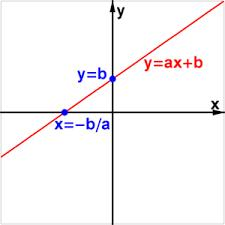# Functions f,g

Find g(1) if g(x) = 3x - x2

Find f(5) if f(x) = x + 1/2

Correct result:

g =  2
f =  5.5

#### Solution:We would be pleased if you find an error in the word problem, spelling mistakes, or inaccuracies and send it to us. Thank you!## Next similar math problems:

• SequenceWrite the first 6 members of these sequence: a1 = 5 a2 = 7 an+2 = an+1 +2 an
• One halfOne half of ? is: ?
• First manWhat is the likelihood of a random event where are five men and seven women first will leave the man?
• Linear systemSolve a set of two equations of two unknowns: 1.5x+1.2y=0.6 0.8x-0.2y=2
• Equations - simpleSolve system of linear equations: x-2y=6 3x+2y=4
• PowersExpress the expression ? as the n-th power of the base 10.
• Volleyball8 girls wants to play volleyball against boys. On the field at one time can be six players per team. How many initial teams of this girls may trainer to choose?
• Two equationsSolve equations (use adding and subtracting of linear equations): -4x+11y=5 6x-11y=-5
• TreesA certain species of tree grows an average of 0.5 cm per week. Write an equation for the sequence that represents the weekly height of this tree in centimeters if the measurements begin when the tree is 200 centimeters tall.
• AverageIf the average(arithmetic mean) of three numbers x,y,z is 50. What is the average of there numbers (3x +10), (3y +10), (3z+10) ?
• Nineteenth memberFind the nineteenth member of the arithmetic sequence: a1=33 d=5 find a19
• TransformerTransformer - U1 = 230 V, N1 = 300, N2 = 1,200, I1 = 4 A. Calculate the transformation ratio, voltage and current in the secondary coil.
• EquationsSolve following system of equations: 6(x+7)+4(y-5)=12 2(x+y)-3(-2x+4y)=-44
• Factory and divisionsThe factory consists of three auxiliary divisions total 2,406 employees. The second division has 76 employees less than 1st division and 3rd division has 212 employees more than the 2nd. How many employees has each division?
• Days2minutesWrite a formula that converts x days to y minutes. The formula is:
• 6 termsFind the first six terms of the sequence. a1 = 7, an = an-1 + 6
• Percentage increaseIncrease number 400 by 3.5%